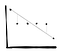Data Knowledge: Science Foundations Explained

A series of shorts diving into algorithms. Let’s start at the beginning.

Linear Regression — a line.

How do we do that mathematically? Well, we want to minimize a distance from a line for all our points — that is what we define as the best fit. This means we need to inspect every point, calculate how far they are from the line, sum them and ‘tada!’.Here the total distance from all points to the line is zero — great a perfect best fit!?

What if you have some huge outliers which are similar distances away but on opposite sides? Intuitively, we know it is wrong but because our calculation simply sums the difference (and that difference can be positive or negative depending if the point is above or below the line) this line’s sum is equal to zero.

We need to minimise the distance but remove the ability to have negative distances. Let’s square the distances and minimise on that.

On this image we can see a new line of fit which is much more like what we had expected. These point have much less distance to the line.

But how do we find the best line?

We could spend all day looking at it and putting a new line on, calculating that line’s distance to all points, or we will use maths.

Let’s say the distance of all points to the line is R. R is the distance from our point to the point the line says it should be, so R=(y − ŷ); where y is the value we have and ŷ is the value our line says it should be. We want that to be squared to account for negative and positive distances — R²=(y − ŷ)² .

Any straight line can be said to have the formula of y = mx + C.

Let’s use this in our formula for R. R²=(y − (mx+C))².

If we rearrange this to solve for m we can get the gradient of the best fitting line. Someone clever did this for us : m = ( n∑xy − (∑x)(∑y) ) / ( n∑x² − (∑x)² )

Plugging in our formula we can get the optimum slope and then find the intercept to get the full formula.